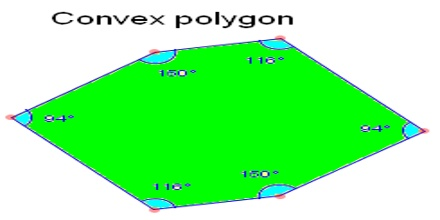Convex polygon is a polygon that has all interior angles less than 180°. Convex polygons are polygons for which a line segment joining any two points in the interior lies completely within the figure. This means that all the vertices of the polygon will point outwards, away from the interior of the shape. Think of it as a ‘bulging’ polygon.Convex Polygon overview

• A line drawn through a convex polygon will intersect the polygon exactly twice. You can also see that the line will divide the polygon into exactly two pieces.
• All the diagonals of a convex polygon lie entirely inside the polygon.
• The area of an irregular convex polygon can be found by dividing it into triangles and summing the triangle’s areas.A convex polygon must satisfy the following properties:

• It has all the interior angles less than or equal to 180°
• It has both the sides joining a vertex either going inside or straight.
• It has each diagonal lying inside or on the boundary of the polygon.
• It has all the vertices pointing outwards.
• If a line segment is drawn through the polygon, then it intersects the boundary of polygon exactly twice.